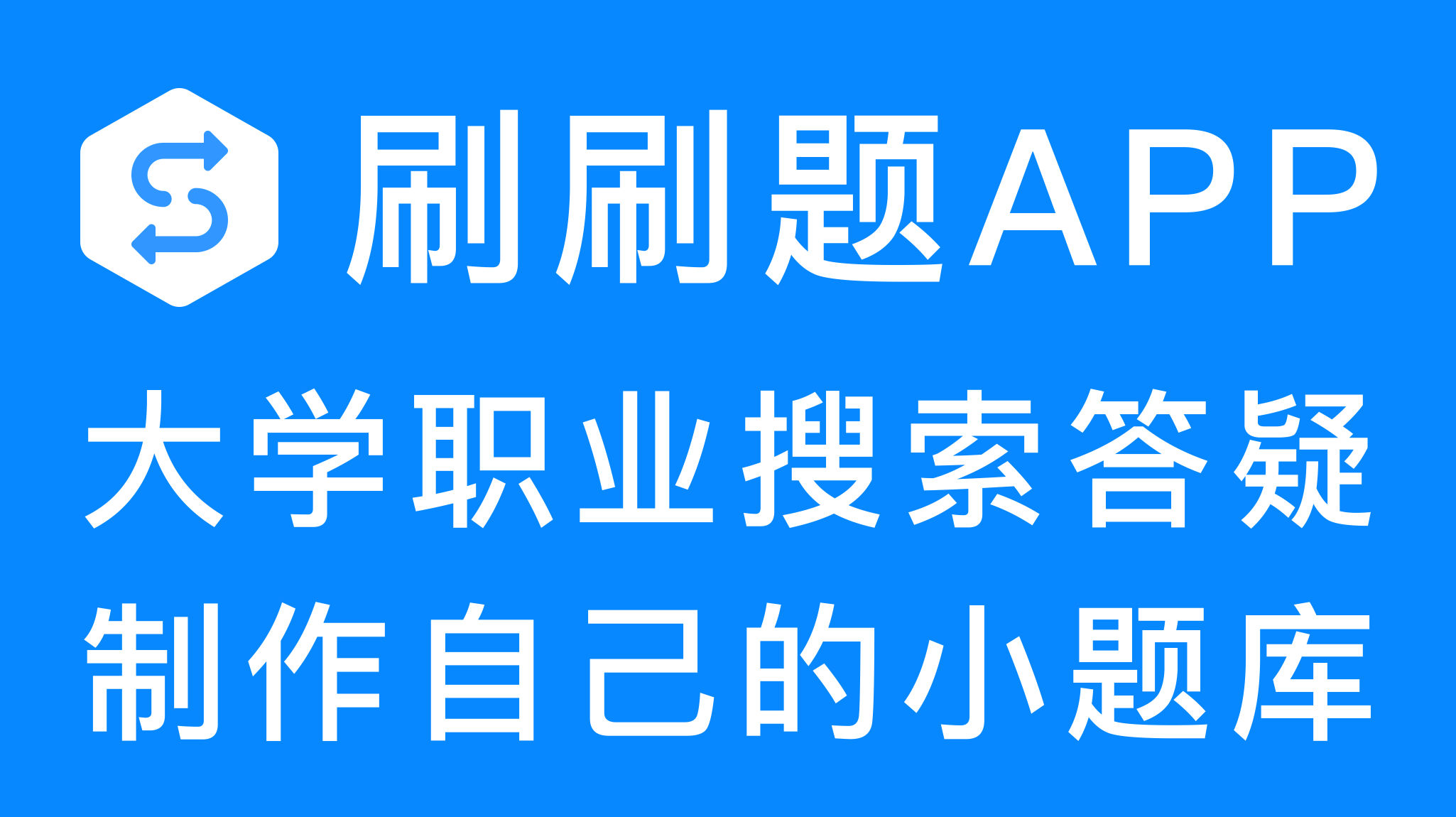WORD模板下载
EXCEL模板下载HR
【多选题】# 监控平台有哪些功能？

A.

B.

C.

D.

E.手机使用分享复制链接新浪微博分享QQ微信扫一扫反馈收藏A.

B.

C.

D.

A.

B.

C.

D.

A.

B.

C.

D.

A.

B.

C.

D.

## 【单选题】有如下事件过程：Private Sub Form_Load() Show Dim Arr() As Variant Arr = Array(1, 3, 5, 7, 9, 11, 13, 15) Call converse(Arr) For i = 0 To 7 Print arr(i); Next iEnd Sub 以上程序的功能是，通过调用Converse过程，将数组Arr中的元素逆序存放并输出...

A.
Private Sub converse(dim a() as Variant ) Dim T For i = LBound(A) To UBound(A) T = a(i) a(i) = a(UBound(A) - i) a(UBound(A) - i) = T Next i End Sub
B.
Private Sub converse(dim a() as Variant) Dim T Dim J As Integer J = (LBound(A) + UBound(A)) / 2 For i = LBound(A) To J T = a(i) a(i) = a(UBound(A) - i) a(UBound(A) - i) = T Next i End Sub
C.
Private Sub converse(a() As Variant) Dim T For i = LBound(A) To UBound(A) T = a(i) a(i) = a(UBound(A) - i) a(UBound(A) - i) = T Next i End Sub
D.
Private Sub converse(a() As Variant) Dim T Dim J As Integer J = Int((LBound(A) + UBound(A)) / 2) For i = LBound(A) To J T = a(i) a(i) = a(UBound(A) - i) a(UBound(A) - i) = T Next i End Sub

A.

B.

C.

D.

A.

B.

C.

D.

E.

## 【多选题】主流跨境电子商务平台包括()

A.
Amazon
B.
eBay
C.
AliExpress
D.
Wish
E.
DHgate
F.
JollyChic【多选题】统一政务网络平台的要求有（）.
A.

B.

C.

D.

【多选题】综合物流共享信息平台的功能包括：（）。
A.

B.

C.

D.

【多选题】支付客户端App是（）的平台。
A.

B.

C.

D.

【单选题】电子商务平台采购管理以( )为准则。
A.

B.

C.

D.

【单选题】有如下事件过程：Private Sub Form_Load() Show Dim Arr() As Variant Arr = Array(1, 3, 5, 7, 9, 11, 13, 15) Call converse(Arr) For i = 0 To 7 Print arr(i); Next iEnd Sub 以上程序的功能是，通过调用Converse过程，将数组Arr中的元素逆序存放并输出...
A.
Private Sub converse(dim a() as Variant ) Dim T For i = LBound(A) To UBound(A) T = a(i) a(i) = a(UBound(A) - i) a(UBound(A) - i) = T Next i End Sub
B.
Private Sub converse(dim a() as Variant) Dim T Dim J As Integer J = (LBound(A) + UBound(A)) / 2 For i = LBound(A) To J T = a(i) a(i) = a(UBound(A) - i) a(UBound(A) - i) = T Next i End Sub
C.
Private Sub converse(a() As Variant) Dim T For i = LBound(A) To UBound(A) T = a(i) a(i) = a(UBound(A) - i) a(UBound(A) - i) = T Next i End Sub
D.
Private Sub converse(a() As Variant) Dim T Dim J As Integer J = Int((LBound(A) + UBound(A)) / 2) For i = LBound(A) To J T = a(i) a(i) = a(UBound(A) - i) a(UBound(A) - i) = T Next i End Sub
【多选题】预警平台预警分为几类。（）
A.

B.

C.

D.

【单选题】个人可以申请的微信公众平台帐号是什么?( )
A.

B.

C.

D.

【多选题】监控平台有哪些功能？
A.

B.

C.

D.

E.

【多选题】主流跨境电子商务平台包括()
A.
Amazon
B.
eBay
C.
AliExpress
D.
Wish
E.
DHgate
F.
JollyChic